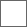• 51回复贴，共1

### 有趣的等值局面现象①：
2子等价于从这六种19个棋子里选1种，只有6种
②：
3子等价于19选2
2子相同：5种
2子不同：6种棋子选2种，C(6,2)=15种

③：
4子等价于19选3
3子全同：只能选兵象，2种

3子全不同：C(6,3)=20种

④：
5子等价于19选4
4子全同：1种
“3+1”：2×5=10
“2+2”：C(5,2)=10
“2+1+1”：5×C(5,2)=50
4子全不同：C(6,4)=15

⑤：
6子等价于19选5
5子全同：1种
“4+1”：1×5=5
“3+2”：2×4=8
“3+1+1”：2×C(5,2)=20
“2+2+1”：C(5,2)×4=40
“2+1+1+1”：5×C(5,3)=50
5子全不同：C(6,5)=64子局面777吧主再研究一下非等值局面吧?例如每方两个棋子的非等值局面跟每方十九个棋子的非等值局面是不是也是一样?不过非等值局面数量比等值局面要多很多，但也是有限的。这是只考虑棋子在棋盘上种类和数量，所以局面总数可以算出来。国际象棋960跟国际象棋原始局面其实是一种局面，因为棋子教量跟种类一样。等会我就暴力箅出两子的非等值局面数。你的数学厉害，能否用公式帮忙算算?2子：6×5÷2=15
3子：20×19÷2=190
4子：47×46÷2=1081
5子：86×85÷2=3655
6子：129×128÷2=8256